# Magnetic Affects on Vehicle Electronics

 Page 1/7 Date 19.05.2018 Size 0.51 Mb. #49116
Magnetic Affects on Vehicle Electronics

Ryan Bussis

Abstract - Magnetics affect the way electronics work in a vehicle. There are certain functions on a vehicle that introduce new magnetics. One of these items is the defroster on a vehicle, due to the current flow that is used to run it. Also, in addition to the defroster a wire will carry the voltage and current needed to run the rear defroster. Also the air conditioning unit will also generate magnetic fields that are strong enough to affect electronics. This paper will focus on what these magnetic fields are, actual measurements and simulation of these magnetic fields. The paper will also focus on the theories used to figure out the magnet forces. Another part of this paper will focus on the magnetization of vehicles. An example of this is when a vehicle travels over subway rails; due to the large amount to magnetic forces in these rails, traveling over it will magnetize a vehicle. This can be a concern for electronics in a vehicle. This aspect of vehicle magnetization will also be focused on. It will be focused on in two areas; the cause of this phenomena, and the effect of this magnetization.
Index Terms – Electric Field, Magnetic Flux, Resolution, Calibration
1. ## Introduction

There are many theories that will apply to the study that will be performed. The theories include magnetic flux density, surface current, uniform sheet current and the Biot-Savart law. These many laws will be needed to understand the affects that magnetics have. There are many magnetic forces in this world, but more specifically there are many forces that lie in your vehicle alone, as well as those that lie outside the vehicle and affect it.

This paper will present an overview and study that was completed in determining the magnetic affects on electronics, with the study focusing on the digital compasses that are in vehicles.

1. ## magnetic laws

The first law is Coulombs law which is the basis of everything that magnetics is built on. If one charge is in a fixed position, and move a second charge slowly around, there exists everywhere a force on this second charge; in
___________________________

Sponsered by Johnson Controls Inc, using US patent 5,878,370

Ryan Bussis with the Department of Engineering at Calvin College, Grand Rapids, MI 49546, USA (e-mail: rbussi88@calvin.edu)

other words the second charge is displaying the existence of a force called a field. The force on it is called Coulombs law: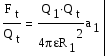Writing this force as a force per unit charge gives: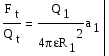The right side of the equation describes a vector field and is called the electric field intensity. The electric field intensity is a vector of force on a unit positive test charge.

Electric field intensity must be measured by the unit newtons per coulomb – the force per unit charge. So the equation for this can be seen below: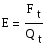Now let us substitute the equation for a point charge, and the following equation can be formed: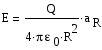Understanding electrical charges is important for the understanding the magnetic fields. Another one of the laws that is important to understand is that

The Biot-Savart Law is the first theory that needs to be known since all the other theories depend on the Biot-Savart Law. The Biot-Savart Law is the magnetic equivalent to the Coulombs Law. The Biot-Savart law basically states that at any point P, the magnitude of the magnetic field intensity produced by the differential element is proportional to the product of the current, the magnitude of the differential length and the sine of the angle lying between the filament and a line connecting the filament to the point P. The equation is as follows: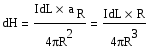Figure 2 shows a model of what the Biot-Savart Law is describing, where dL is the infinitesimal length of the conductor carrying electric current. R is the unit vector specifying the direction of the vector distance from the current to the field point. dB is the magnetic field contribution from the current element, and dL is the relationship between the magnetic field and its current source.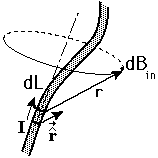Figure 1 – Biot-Savart Law 
The law of Biot-Savart is sometimes called Ampere’s law for the current element. In some aspects the Biot-Savart law is a lot like Coulomb’s law when it is written for a differential element charge. Both of these laws show an inverse square law dependence on distance, and a linear relationship between source and field, the only difference is the direction of the field. This law can be applied in many locations. One of those is the infinitely long wire. The direction of the current is found by using the right hand rule. Point your thumb in the direction of the current flow and your fingers indicate the direction of the circular magnetic fields around the wire. The Biot-Savart law can also be expressed in terms of distribution sources such as current density J and surface current density, which will be discussed later in the paper.

Magnetic flux density is measured in webers per square meter, or a newer unit adopted by the International System of Units called the tesla. The old unit is the gauss where it equals 10,000 G to 1 tesla, which also equals 1 Wb/m2. Magnetic flux is figured out by the equation B = μ* H. Where H is the integral of the equation in figure 1, and μ is the constant 4π x 10-7 H/m as defined for free space. A figure showing what magnetic flux is can be seen below in Figure 3.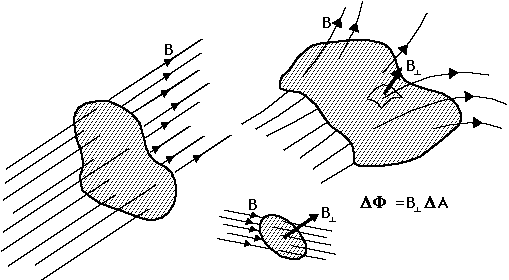Figure 2 – Magnetic flux 

The magnetic flux density vector B is a member of the flux density family of vector fields which compares the laws Biot-Savart and Coulomb, thus the relation of B and E. If B is measured in teslas or webers per square meter, then magnetic flux should be measured in webers. Electric flux and Gausses law state that the total flux passing through any closed surface is equal to the charged enclosed.

The charge Q is the source of the lines of electric flux and these lines begin and terminate on positive and negative charges. However, no source has been discovered for the lines of magnetic flux. For lets say an infinitely long straight filament carrying a direct current I the H field formed concentric circles about the filament. Since we know the equation for magnetic flux B is in the same form. For this reason Gausses law is:

Surface Current flows in a sheet of infinite thickness and the current density is measured in amperes per square meter. Surface current density is measured in amperes per meter width. Figure 4 is showing how surface current theory will be used for the purpose of this paper.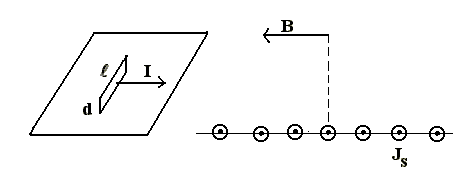Figure 3 – Sheet current theory 

If there are a large number of wires running parallel to each other within a confined distance, like on the right side of figure 4, then it can be considered an infinitely thin sheet of current in space. A defroster on a vehicle is an example of this. With around 20 wires that are lined up inches from eachother they would be considered an infinite sheet of current, sine the power and current go in one end and come out one place on the other.